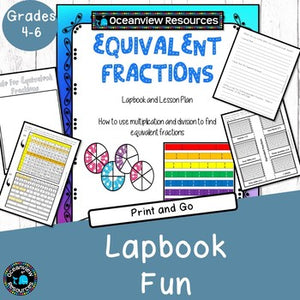Oceanview Education and Teaching Supplies

# Equivalent Fractions - Lapbooks and Explorations

Regular price \$5.00 \$0.00 Unit price per
Tax included at checkout

Many simple equivalent fractions are easy to find on a regular multiplication chart. Many students struggle as they try to learn the many steps that it takes to find equivalent fractions. When you look left to right on the chart you are viewing the multiples of a number.

When you view two different rows of multiplies you are viewing equivalent fractions. For example using the 1's and 2's. Look at the two rows as if they were fractions.: 1/2 = 2/4 = 3/6 = 4/8 and so on.

You can split rows apart. Look at the rows for 1's and 3's. 1/3 = 2/6 = 3/9 = 4/12 and so on As your students understand how to work the chart, encourage them to explore other ways of finding equivalent fractions.

It makes it much easier for them to "see" the equivalent fractions for a number and the students even start memorizing the most common equivalent fractions. *****Now for the most important part.

Encourage your students them to make a multiplication chart from their multiplication facts and have them practice often. It should take less than 5 minutes. Not only can they use the chart for checking fraction, but they can use it as they do multiplication and division problems too.

It is all about using their prior knowledge and applying it to new tasks.
You will receive a 13 page PDF complete with 2 samples of lapbooks